### MEMO 2011 ekipno problem 7

Kvaliteta:
Avg: 1,0
Težina:
Avg: 4,5
Let$A$ and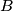$B$ be disjoint nonempty sets with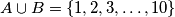$A \cup B = \{1, 2,3, \ldots, 10\}$. Show that there exist elements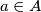$a \in A$ and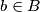$b \in B$ such that the number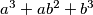$a^3 + ab^2 + b^3$ is divisible by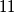$11$.
Izvor: Srednjoeuropska matematička olimpijada 2011, ekipno natjecanje, problem 7